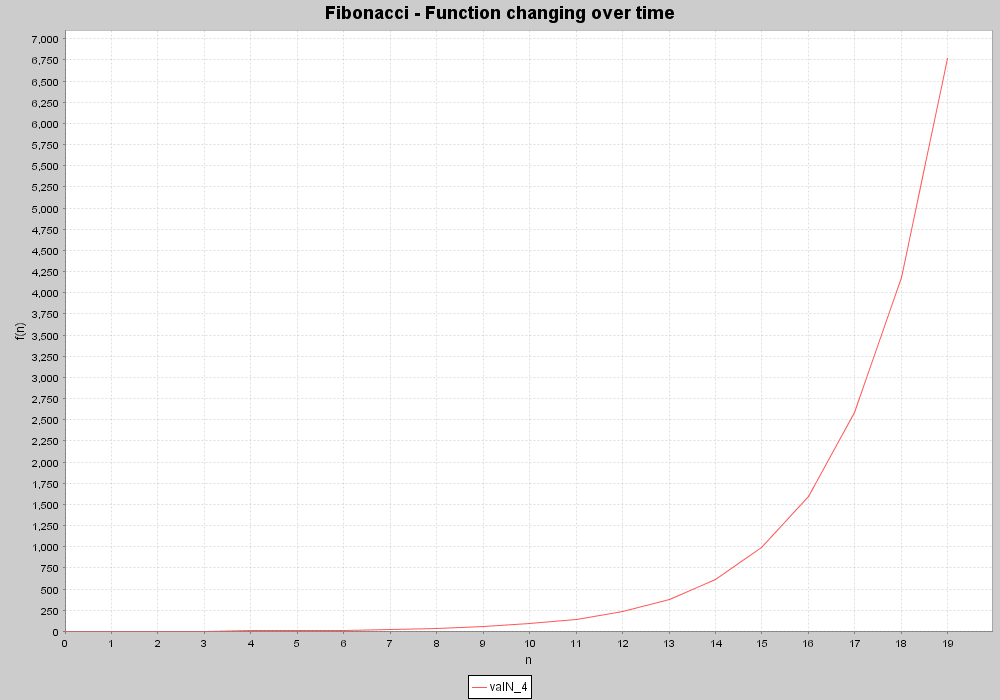# Dynamical System - Fibonacci - Xholon App

## What is it

This model implements a dynamical system that creates the Fibonacci series, where each successive number is the sum of the two preceding numbers. This demonstrates how to model dynamical systems using Xholon.

## How to use it

Run it:

1. Run the Java application through the Xholon GUI (org.primordion.xholon.app.Xhn.java), and select File --> Open --> dynsys --> Fibonacci --> Fibonacci_xhn.xml.
2. Expand the Controller node in the tree.
3. Press the Start node.
4. If you have JFreeChart installed you should see a chart showing the Fibonacci series over time.
5. If you don't have JFreeChart installed, then change the value of UseDataPlotter to "gnuplot", and look at the results that are saved to a .csv file in the statistics folder. If you have gnuplot installed, it can read the .plt script in the statistics folder to produce a displayable .png file from the .csv file, that should look similar to the one below.
```

```## Things to notice

Since the default values are ints, the output is valid only until n=47. See the next section 'Things to try' for instructions on how to extend this.

## Things to try

Extend the maximum range of the application by converting the java variables to doubles. First, select the desired 'n' value by editing Fibonacci_xhn.xml (param name="MaxProcessLoops" value="21"/>)

Then edit XhFibonacci.java. Declare getVal_int() and and getVal as doubles, and change each occurrence of getVal_int() to getVal_double(). In the act() method change ((XhFibonacci)port[P_DEPVAR]).setVal(0) to ...setVal(0.0), and ((XhFibonacci)port[P_DEPVAR]).setVal(1) to ...setVal(1.0)

Note that for n values greater than 68 JFreeChart will no longer display the range values clearly. You can still get a sense of the magnitude of the result for large n's by right clicking JFreeChart to see how it has autoscaled the Y-axis maximum value.

## Xholon and Ealontro features

Each successive number is a new xholon that has been instantiated as a result of the preceding two numbers (xholons) interacting with each other via their ports.